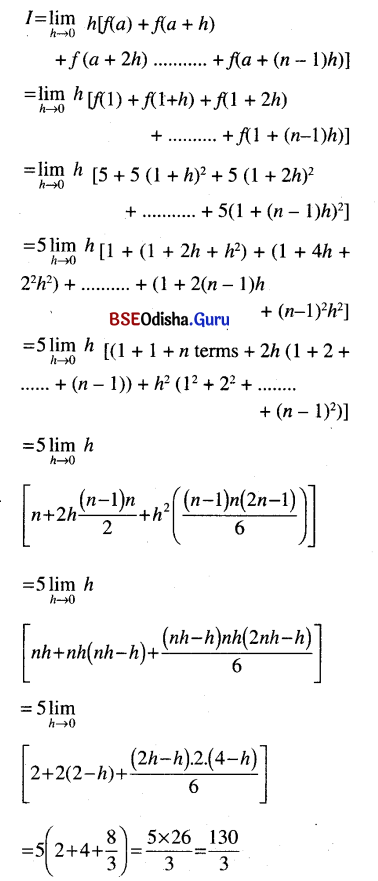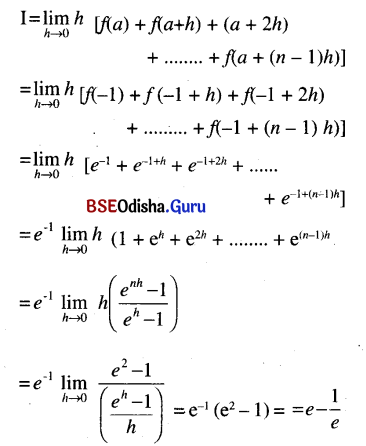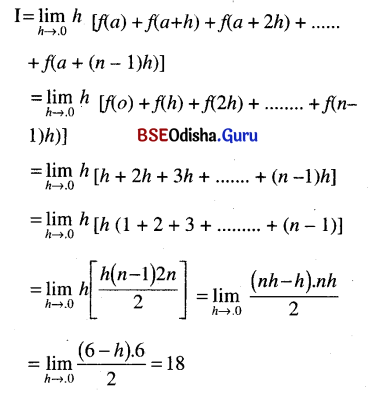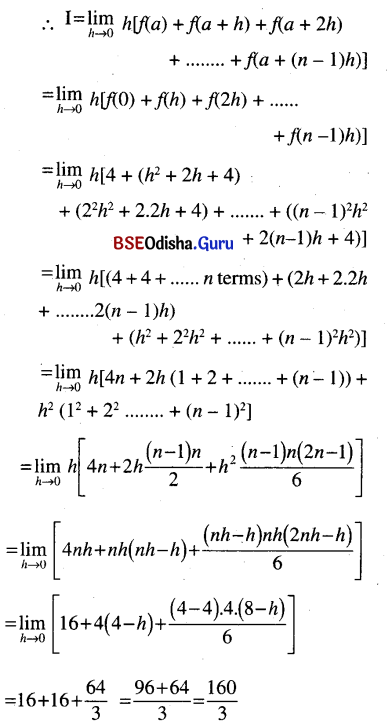# CHSE Odisha Class 12 Math Solutions Chapter 9 Integration Ex 9(i)

Odisha State Board Elements of Mathematics Class 12 Solutions CHSE Odisha Chapter 9 Integration Ex 9(i) Textbook Exercise questions and Answers.

## CHSE Odisha Class 12 Math Solutions Chapter 9 Integration Exercise 9(i)

Evaluate the following Integrals.
Question 1.
I = $$\int_1^3$$5x2 dx
Solution:
Here a = 1, b = 3, f(x) = 5x2
⇒ nh = b – a = 3 – 1 = 2Question 2.
I = $$\int_{-1}^1$$ex dx
Solution:
Here a = -1, b = 1, f(x) = x, nh = b – a = 2Question 3.
I = $$\int_0^6$$x dx
Solution:
Here a = 0, b = 6, f(x) =x, nh = b – a = bQuestion 4.
I = $$\int_0^4$$(x2 + 2x + 4) dx
Solution:
Here a = 0, b = 4, f(x) = x2 + 2x + 4 and nh = b – a = 4